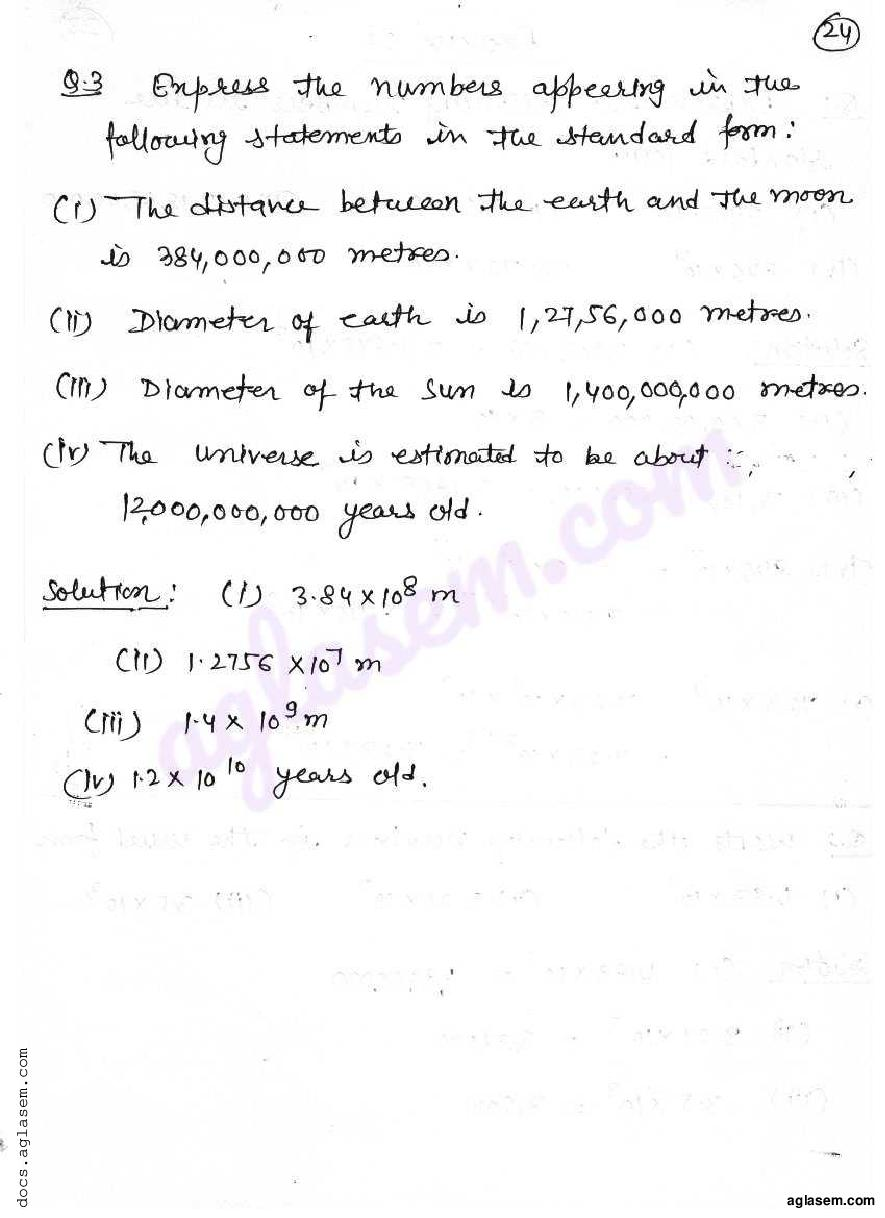# RD Sharma Solutions Class 7 Chapter 6 Exponents Exercise 6.3

Here you can get free RD Sharma Solutions for Class 7 Maths Chapter 6 Exponents Exercise 6.3. All RD Sharma Book Solutions are given here exercise wise for the chapter Exponents. RD Sharma Solutions are helpful in the preparation of several school level, graduate and undergraduate level competitive exams. Practicing questions from RD Sharma Mathematics Solutions for Class 7 Chapter 6 Exponents is proven to enhance your math skills.

 Class: Class 7th Chapter: Chapter 6 Exercise: Exercise 6.3 Name: Exponents

## RD Sharma Solutions Class 7 Chapter 6 Exponents Exercise 6.3

RD Sharma Class 7 Solutions Chapter 6 for Exponents Exercise 6.3 are given below.

RD Sharma Solutions Class 7 Chapter 6 Exponents Exercise 6.3